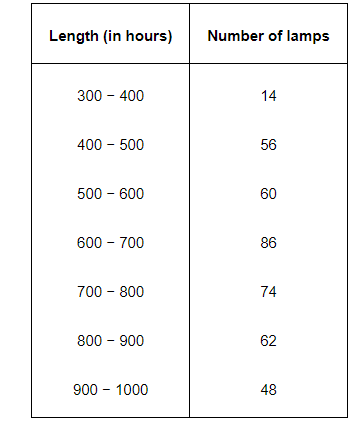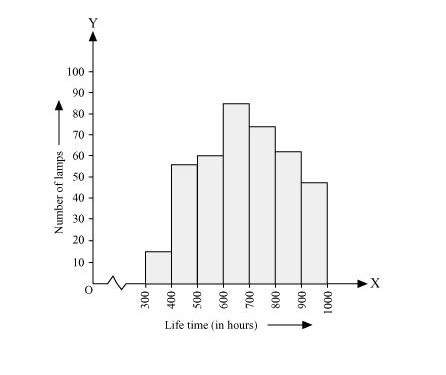# The following table gives the life times of neon lamps:Question.

The following table gives the life times of neon lamps:(i) Represent the given information with the help of a histogram.

(ii) How many lamps have a lifetime of more than 700 hours?

Solution:

(i) By taking life time (in hours) of neon lamps on x-axis and the number of lamps on y-axis, the histogram of the given information can be drawn as follows.Here, 1 unit on y-axis represents 10 lamps.

(ii) It can be concluded that the number of neon lamps having their lifetime more than 700 is the sum of the number of neon lamps having their lifetime as 700 − 800, 800 − 900, and 900 − 1000.

Therefore, the number of neon lamps having their lifetime more than 700 hours is 184. (74 + 62 + 48 = 184)